The inverse of 3 x 3 matrix with determinants and adjugate

The inverse of 3 x 3 matrix with determinants and adjugate

In this lesson, you will learn the long way of computing the inverse of a 3 x 3 matrix. This method requires 4 steps. The first step is the matrix of minor. Each entry in the matrix is a 2 x 2 matrix that is not in that entry's row or column. The second step is the adjugate. This requires you to take your matrix of minors and changing the signs of certain entries depending on the negative signs that appear in the checkerboard. The third step is to transpose. This requires you to switch all the rows and make them into columns. The last step is to multiply your transposed matrix by 1 over the determinant of the original matrix (scalar multiplication). All of these steps should now give you the inverse.

Lessons

This method is the long way of computing the inverse of a 3 x 3 matrix. To do this, we need to go through 4 steps:

1) The Matrix of Minors
3) Transpose
4) Multiply by $\;\frac{1}{determinant\; of\; original\; matrix}$

Once we apply these steps, then we will find the inverse.
• Introduction
The Inverse of 3 x 3 Matrix Overview:
a)
The Matrix of Minors

b)

c)
Transpose

d)
Multiply by $\;\frac{1}{determinant\; of\; original\; matrix}$

• 1.
Finding the Matrix of Minors
You are given that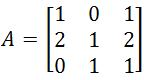. Find the Matrix of Minors

• 2.
Finding the Adjugate Matrix
You are given that the matrix of minors is. Find the Adjugate matrix.

• 3.
You are given that the Adjugate Matrix is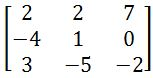. Transpose this matrix.
You are given that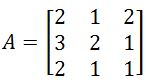. The transposed adjugate of this matrix is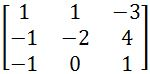. Find the inverse of $A$.
You are given that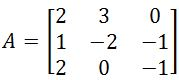. Find the inverse of this matrix
You are given that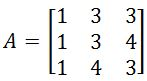. Find the inverse of this matrix